# 于OneFlow实现Unfold Fold算子

### 从卷积层说起¶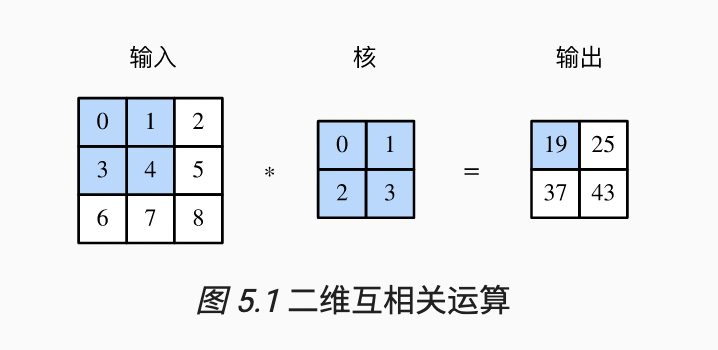from mxnet import autograd, nd
from mxnet.gluon import nn

def corr2d(X, K):  # 本函数已保存在d2lzh包中方便以后使用
h, w = K.shape
Y = nd.zeros((X.shape - h + 1, X.shape - w + 1))
for i in range(Y.shape):
for j in range(Y.shape):
Y[i, j] = (X[i: i + h, j: j + w] * K).sum()
return Y


### 初见img2col¶https://github.com/microsoft/AI-System/blob/main/docs/SystemforAI-4-Computer%20architecture%20for%20Matrix%20computation.pdf 这是微软的AISystem仓库对应的章节，强烈推荐大家去学习（本人鸽了好久没看完）

### 理解img2col¶

• 假设输入特征图为(N, Cin, H, W)，卷积核参数为(K, K, Cin, Cout), 输出特征图的长宽为(Oh, Ow)

img2col算法是一种用空间换时间的做法，将输入进行变换后，显然它所占用的显存空间变大了，好处则是可以借助矩阵乘，快速地完成卷积运算。

### img2col源码¶

darknet的img2col其实是搬运自caffe的，我们这里为了方便理解，以简单的CPU版本为例子，且不考虑batch维度。

def darknet_img2col(data, channels, height, width, ksize, stride, pad):
out_h = int((height + 2*pad - ksize) / stride) + 1
out_w = int((width + 2*pad - ksize) / stride) + 1

channels_cols = channels*ksize*ksize
out_shape = (channels_cols, out_h*out_w)
elem_cnt = out_shape * out_shape

out_array = np.zeros(shape=elem_cnt, dtype=np.float32)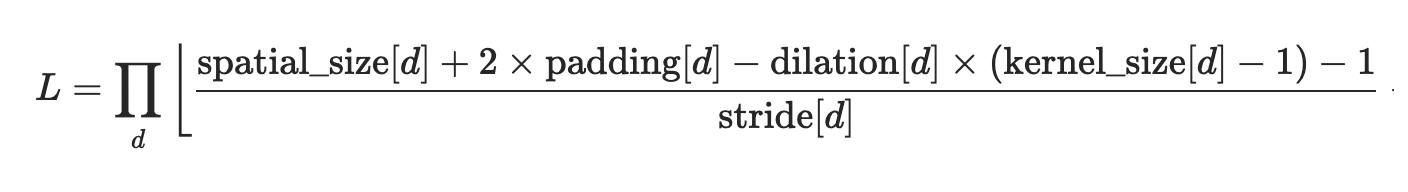channel_cols则是之前我们提到的，img2col会把第二个维度变换为C_in*K*K

    for c in range(channels_cols):
# 分别计算一个k*k的窗口内，h，w的偏移量
kh_offset = (c // ksize) % ksize
kw_offset = c % ksize
# 计算当前处理的通道index
c_im = c // ksize // ksize


        for h in range(out_h):
for w in range(out_w):
im_row = kh_offset + h * stride
im_col = kw_offset + w * stride
index = (c * out_h + h) * out_w + w


im_row的计算方式逻辑是：当前处理的输入元素窗口起始点：即h*stride，然后加上窗口内的kh_offset偏移量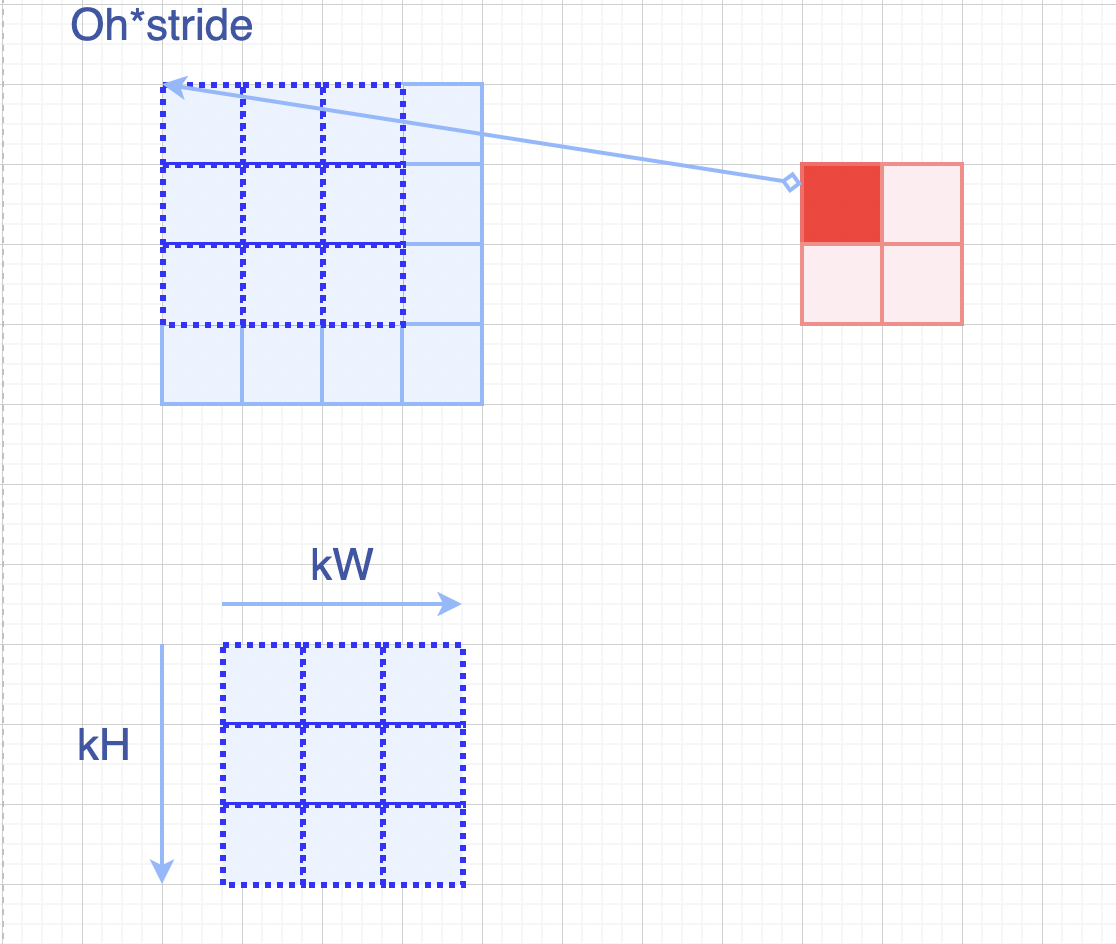当前channel*Oh*Ow + 当前h*Ow + 当前w


                out_array[index] = im2col_get_pixel(data, height, width, c_im, im_row, im_col,  pad)

out_array = np.reshape(out_array, out_shape)

return out_array


img2col_get_pixel是一个合法取元素的函数，如果出现越界范围（比如小于0，或者大于Oh），那么就是取到padding的部分，此时我们返回0。

def im2col_get_pixel(im, height, width, channel, row, col, pad):
if row < 0 or col < 0 or row >= height or col >= width:
return 0
return im[int(col + width * (row + height * channel))] # batch*w*h*c + width*height*channel + width*row + col


x = np.arange(1, 10).astype(np.float32)
out = darknet_img2col(x, channels=1, height=3, width=3, ksize=2, stride=1, pad=0)


[[1. 2. 4. 5.]
[2. 3. 5. 6.]
[4. 5. 7. 8.]
[5. 6. 8. 9.]]


### col2img¶

col2img则是img2col的逆过程，有兴趣的读者可以参考下这个博客：

https://blog.csdn.net/caicaiatnbu/article/details/102626135

### OneFlow版本的Unfold¶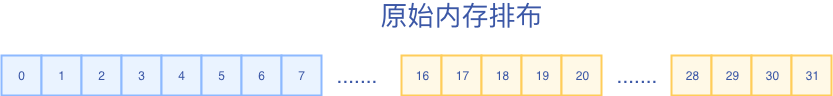in_index_helper = NdIndexOffsetHelper<INDEX_T, kInputNDim>(input_dims); // 格式为(N, C, H, W)
out_index_helper = NdIndexOffsetHelper<INDEX_T, kOutputNDim>(output_dims); // 格式为(N, C, Kh, Kw, Oh, Ow)


// index_a format: (N, C, di, hi, wi, db, hb, wb) or (N, di, hi, wi, db, hb, wb, C)
// index_b format: (N, C, D, H, W) or (N, D, H, W, C)
// return: true indicates out-of-bound, otherwise in-bound
template<typename INDEX_T, int NDIM, int SDIM>
OF_DEVICE_FUNC bool UnfoldIndexTransform(const UnfoldParams<INDEX_T, NDIM, SDIM>& params,
const INDEX_T* index_a, INDEX_T* index_b) {
// batch dim index transform
index_b = index_a;
// channel dim index transform
using ParamType = UnfoldParams<INDEX_T, NDIM, SDIM>;
index_b[ParamType::kInputChannelDim] = index_a[ParamType::kOutputChannelDim];
// spatial dim index transform

// D,H,W spatial dim index transform
for (int64_t d = 0; d < NDIM; ++d) {
INDEX_T idx = index_a[SDIM + NDIM + d] * params.stride[d]
+ index_a[SDIM + d] * params.dilation[d] - params.padding[d];
if (idx < 0 || idx >= params.dims[d]) return true;
index_b[SDIM + d] = idx;
}
return false;
}

• 模板参数 INDEX_T表示Index的数据类型（可以有int32_t, int64_t），NDIM表示处理几维数据（这里我们是2维），SDIM则是决定通道维所在位置，SDIM=1是NHWC格式，SDIM=2则是NCHW格式（这里我们取2）
• 输入参数 index_a表示输出的NdIndexHelper，index_b则表示的是输入的NdIndexHelper

Oh*stride_h + kh*dilation_h - padding_h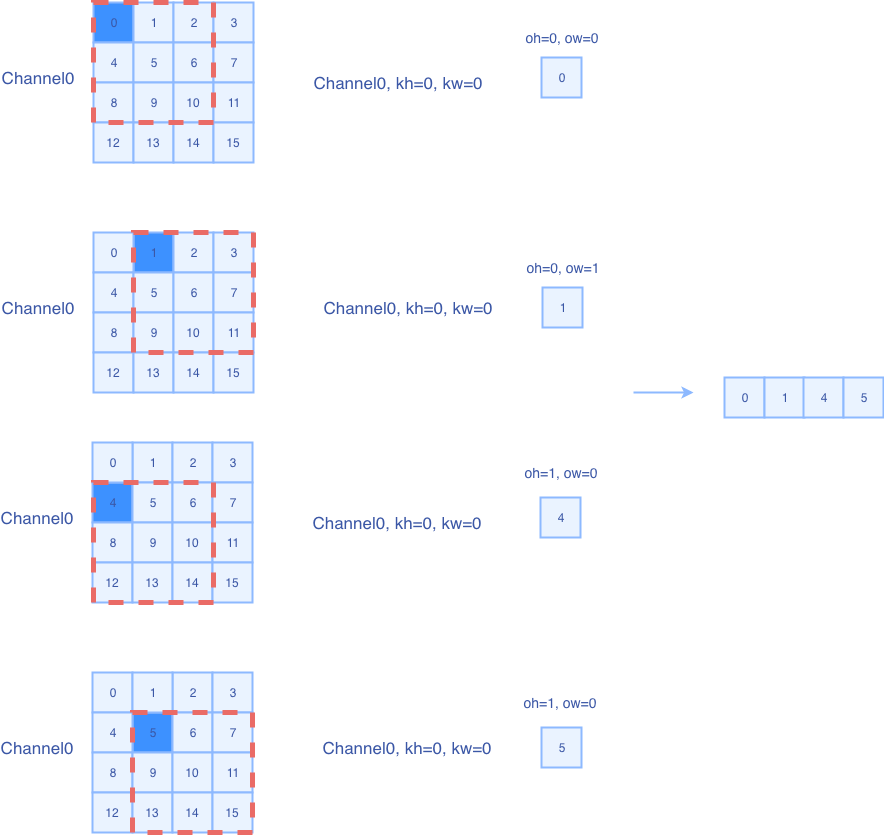template<typename T, typename INDEX_T, int NDIM, int SDIM>
__global__ void CudaUnfoldForward(UnfoldParams<INDEX_T, NDIM, SDIM> params, const T* in, T* out) {
CUDA_1D_KERNEL_LOOP_T(INDEX_T, out_offset, params.out_elem_cnt) {
using ParamType = UnfoldParams<INDEX_T, NDIM, SDIM>;
INDEX_T in_index[ParamType::kInputNDim] = {0};
INDEX_T out_index[ParamType::kOutputNDim] = {0};
params.out_index_helper.OffsetToNdIndex(out_offset, out_index);
if (!UnfoldIndexTransform<INDEX_T, NDIM, SDIM>(params, out_index, in_index)) {
INDEX_T in_offset = params.in_index_helper.NdIndexToOffset(in_index);
out[out_offset] = in[in_offset];
} else {
}
}
}

• 首先根据offset来计算当前处理输出元素的NdIndex
• 然后判断UnfoldIndexTransform该方法的返回值
• 如果为false，则说明我们可以取到输入元素，将其index转换为1d的offset，并赋值给输出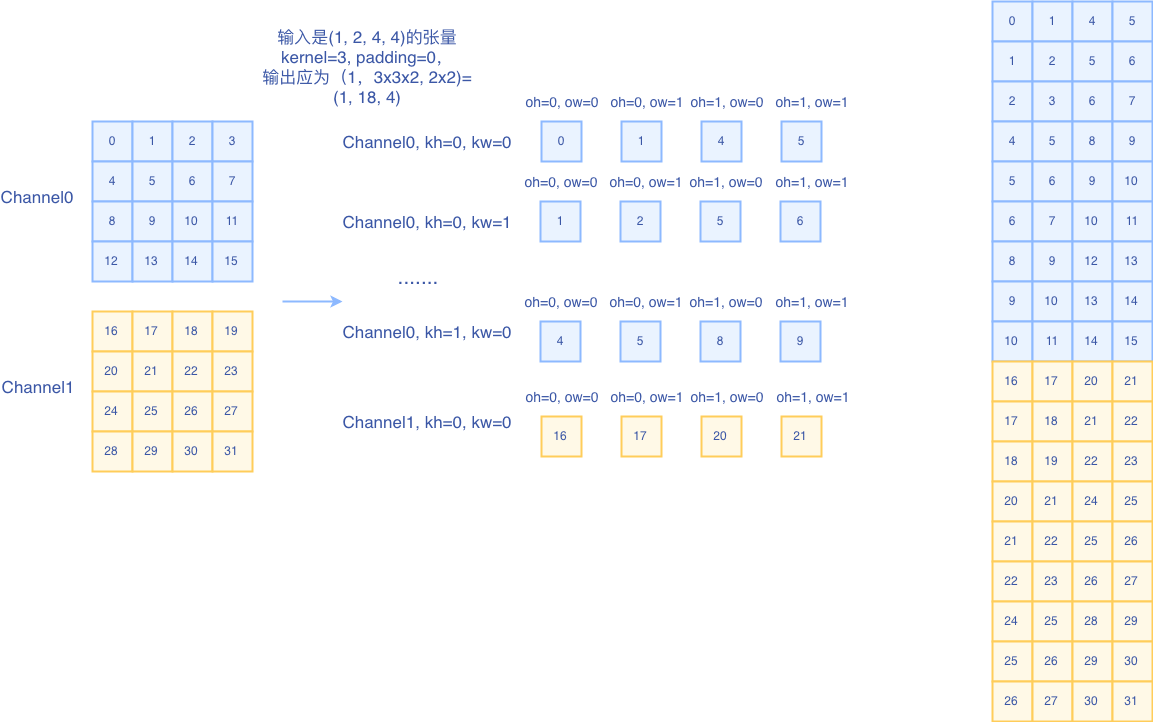### OneFlow版本的Fold¶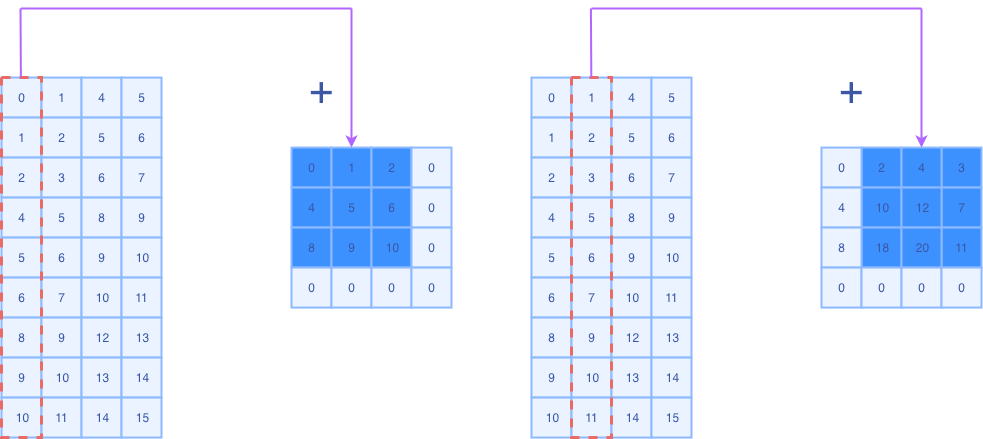Fold就是将每一列填充回到kxk的地方

template<typename T, typename INDEX_T, int NDIM, int SDIM>
__global__ void CudaFoldForward(FoldParams<INDEX_T, NDIM, SDIM> params, const T* input_ptr,
T* output_ptr) {
CUDA_1D_KERNEL_LOOP_T(INDEX_T, in_offset, params.in_elem_cnt) {
using ParamType = FoldParams<INDEX_T, NDIM, SDIM>;
INDEX_T in_index[ParamType::kInputNDim] = {0};
INDEX_T out_index[ParamType::kOutputNDim] = {0};
params.in_index_helper.OffsetToNdIndex(in_offset, in_index);
if (!FoldIndexTransform<INDEX_T, NDIM, SDIM>(params, in_index, out_index)) {
INDEX_T out_offset = params.out_index_helper.NdIndexToOffset(out_index);
} else {
continue;
}
}
}


### 小结¶

darknet版本(Caffe同款)是比较直观的帮助新手入门的img2col算法，可以结合那两篇csdn博客来理解整个过程。